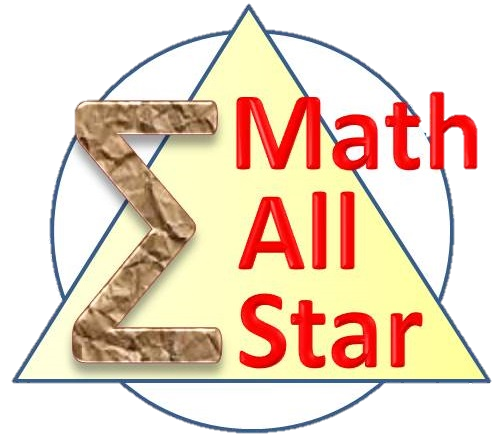#### Pre-assessment - Practical CalculusProblem - 4516

Compute $$\lim_{x\to 4}\frac{3-\sqrt{x+5}}{x-4}$$

Problem - 4517

Is the $y=\frac{1}{x}$ a continuous function?

Problem - 4518

Show that $$\lim_{x\to 0}\ \frac{x}{\sin{x}}=1$$

Problem - 4521

Show that the limit of $f(n)=\left(1+\frac{1}{n}\right)^n$ exits when $n$ becomes infinitely large.

Problem - 4530

Compute the limit of the power series below as a rational function in $x$:

$$1\cdot 2 + (2\cdot 3)x + (3\cdot 4)x^2 + (4\cdot 5)x^3 + (5\cdot 6)x^4+\cdots,\qquad (|x| < 1)$$

Problem - 4548

Determine the values of $\alpha$ and $\beta$ such that

$$\lim_{n\to\infty}\frac{n^{\alpha}}{n^{\beta}-(n-1)^{\beta}}=2020$$

Problem - 4550

Evaluate

$$\int_0^1 \sqrt{1-x^2} d{x}$$

Problem - 4553

Which one of the numbers below is larger?

$$\int_0^{\pi} e^{\sin^2x}dx\qquad\text{and}\qquad \frac{3\pi}{2}$$

Problem - 4558

For $n=1, 2,\dots$, let $x_n=\displaystyle\sum_{k=n+1}^{9n}\frac{k}{9n^2 + k^2}$. Find the value of $\displaystyle\lim_{n\to\infty}x_n$.

Problem - 4627

Compute $$\int\frac{x+1}{x^2+x+1}dx$$